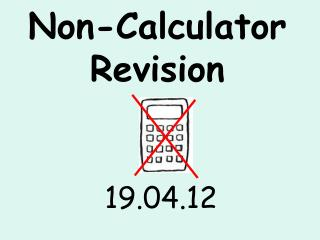DownloadDownload PresentationNon-Calculator Revision

# Non-Calculator Revision

Download Presentation## Non-Calculator Revision

- - - - - - - - - - - - - - - - - - - - - - - - - - - E N D - - - - - - - - - - - - - - - - - - - - - - - - - - -
##### Presentation Transcript

1. Non-Calculator Revision 19.04.12

2. What are we doing? • You will need a partner for this activity. • We will cover 9 topics: number, FDP, addition and subtraction, multiplication and division, measures, 2d shape, 3d shape, angles and handling data. • You will be given 4 minutes to work independently on each section. You will then have a further 2 minutes to share answers with your partner. You must decide on an answer as a team, explaining to your partner how you reached the answer. • Points will be added for sensible behaviour and excellent explanations, but beware, points can be deducted for calling out or not focusing! • PLEASE SELECT A PARTNER, A PIECE OF PAPER, A WHITEBOARD AND A TEAM NAME!

3. Properties of number • What is 16379 rounded to the nearest hundred? • What is 382 ÷ 100? • The temperature in NSC was 7º and decreased by 11º overnight. What is the new temperature? • Complete this sequence: 0.35 0.48 0.61 0.74…? • Which of the following numbers are 1 less than a multiple of 7: 18 27 48 75 13 • What is the first prime number after 14?

4. Fractions, decimals and percentages • ¾ is equivalent to ?/20 • What is 18/42 in its simplest form? • Arrange these fractions in ascending order: 5/8 ¾ ½ 9/16 • Change 27/8 to a mixed number • Change 6 7/11 to an improper fraction • Arrange these decimals in ascending order: 1.05 1.3 1.21 1.006 • What is 3/10 as a decimal and a percentage? • Find 4/5 of 60 • Deduct 15% from £42 • Lenny has 3 green marbles to every 4 red marbles. If he has 20 red marbles, how many green marbles does he have?

5. Addition and Subtraction • Sally has £3159 in her bank account. She withdraws £1374. how much is left in the account? • What is 1.25 added to 14.6? • 7.1 - ? = 2.8 • Find the difference between 729 and 42.6 • Jon walks for 1.6km. He rests for a few minutes then walks a further 1400m. How far has he travelled?

6. Multiplication and Division ÷ • 15 x 99 = • 2.38 x 3 = • How many 6s are in 456? • There are 28 nails in a bag, and 35 bags in a box. How many nails are there in 2 boxes? • A bus holds 14 passengers. How many buses are needed for 87 passengers? • What is the product of 1574 and 8?

7. 12mm 6mm 4cm 4cm 3cm 7cm Measures • 1.9l = ? ml • A ribbon is 1.8m long. 136cm is cut off. How long is the ribbon now? • I start watching a tv programme at 7.45. It lasts for 40 minutes. What time does it finish? • What length is the other side of a rectangle if one side is 8cm and the area is 72cm²? • Find the area and perimeter of these rectangles:

8. 2D Shapes • Which of the following are quadrilaterals? • What shape is this: • Describe right angled, isosceles and scalene triangles.

9. 3D Shapes • How many vertices on a triangular based prism? • How many faces on a cuboid? • How many edges on a square based pyramid?

10. x 27º Angles • Calculate the missing angles: (Or find x Alex C!)

11. Handling Data The number of goals scored in netball matches by NSC: • 1 0 2 3 4 0 1 2 4 1 5 0 1 3 Find the mean, median, mode and range.